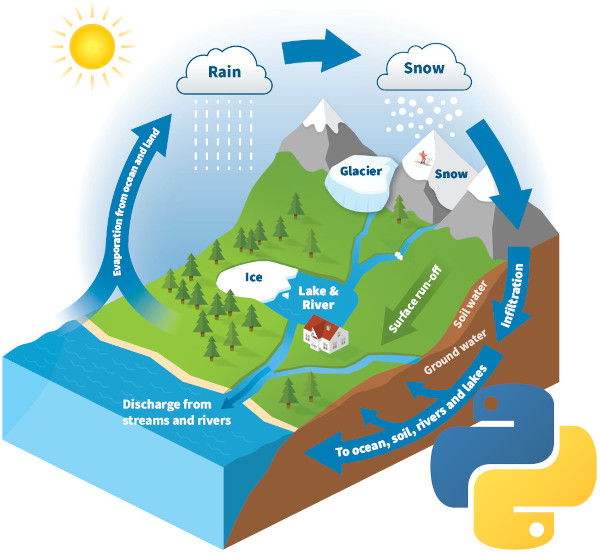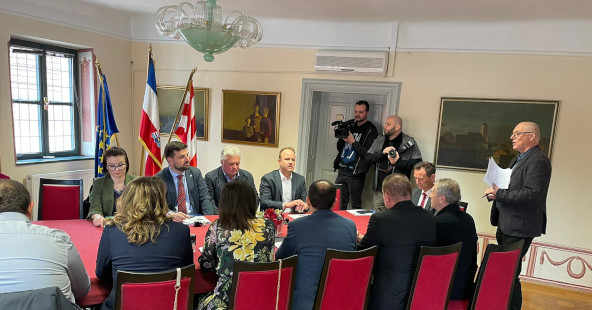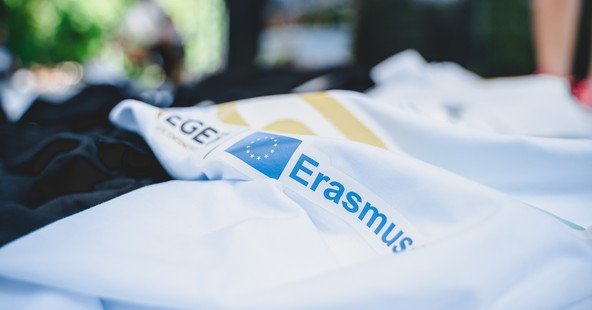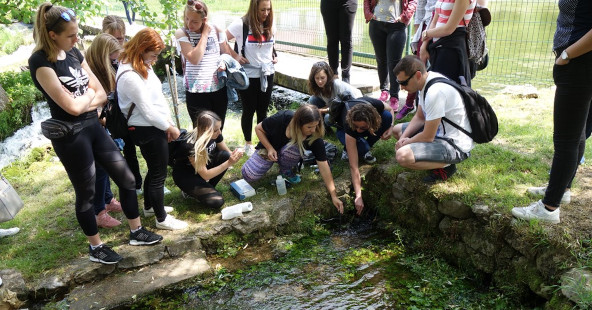## Basic of Python programming through Hydrology###### Category: Projekti

Through this summer school you will learn the first steps of programming in the Python programming language, through interesting examples from Hydrology.

Teacher: DSc Karlo Leskovar

Target group: technical college students, high school seniors, anyone who wants to start programming

Short description:
The goal of this summer school is to introduce students to the basics of Python programming and to provide a framework on how to easily replace spreadsheet programs. It will be shown how to load, analyse and print the actual hydrological data in a diagram. In addition, a basic prediction model based on linear regression, polynomial regression, the K-nearest neighbours method, and Decision-Tree methods will be developed. The developed models will be evaluated to determine their accuracy.

### Curriculum:

1. A brief introduction to Hydrology and the Python programming language
• What is Hydrology?
• What is Python?
• Installing the necessary tools - setting up the Python development environment
• Jupyter Notebook and Anaconda (cell types in the Jupyter Notebook, introduction to the interface)
• Starting the first program (script)
2. Basic data types in Python
• Strings (words, letters)
• Numbers (integes, floats, doubles)
• Variables
3. Data Structures
• Lists
• Dictionaries
• Tuples
4. Control flow and loops
• If-else
• for loop
• while loop
• Functions
5. NumPy i Pandas libraries – working with arrays and table data - loading files (.csv, .xlsx)
6. Basics of diagram plotting– matplotlib.pyplot
• Line plots
• Scatter plots
• Bar plots
• Combined plots
7. Fundamentals of machine learning - regression methods - scikit-learn library
• Linear regression
• Polynomial regression
• K-neighbours
• Decision Tree
• Evaluation methods – MSE, MAE, r2### Potpisan ugovor o projektu „One Sun Connecting North and South“### Natječaj - radna mjesta za docente